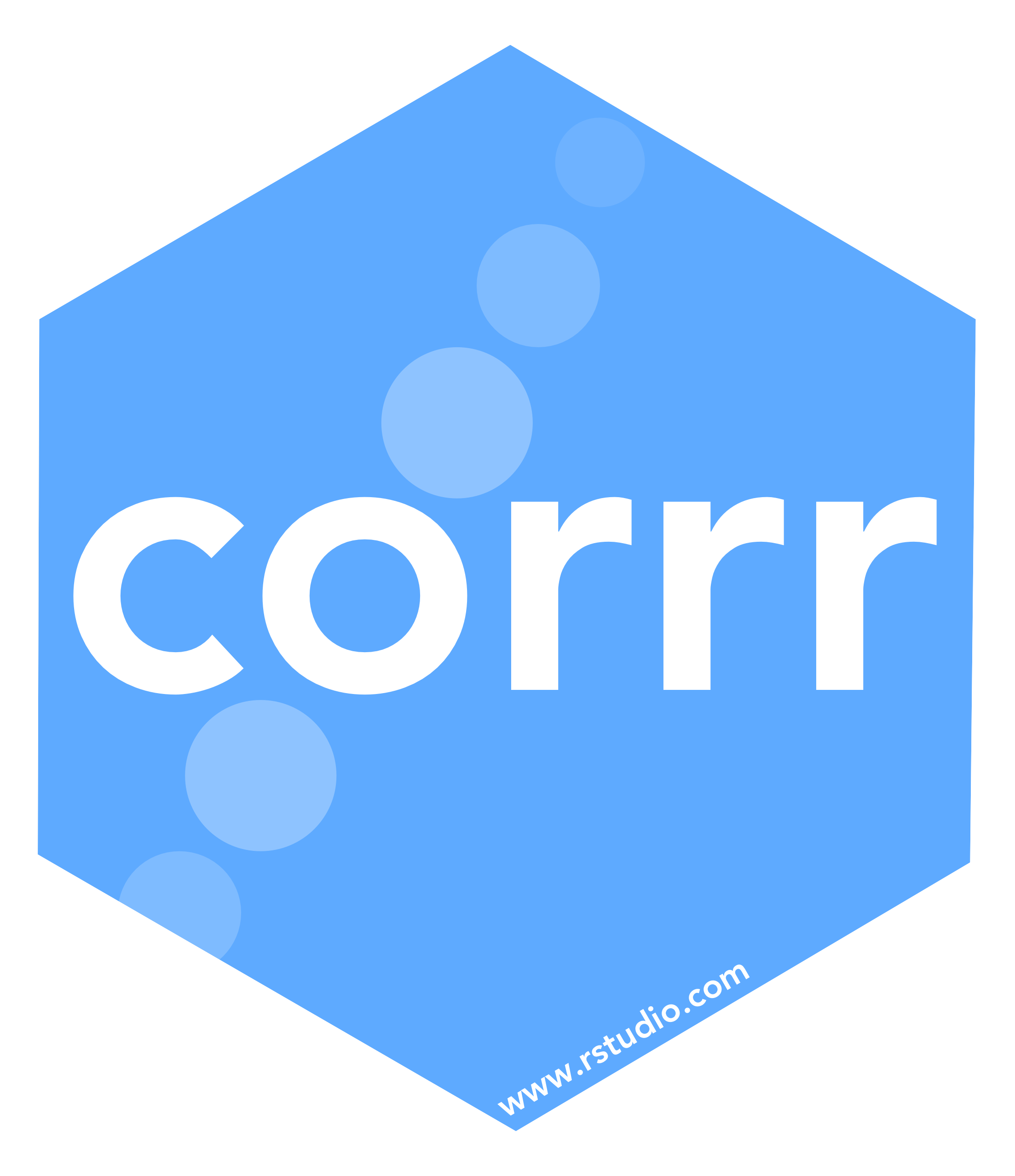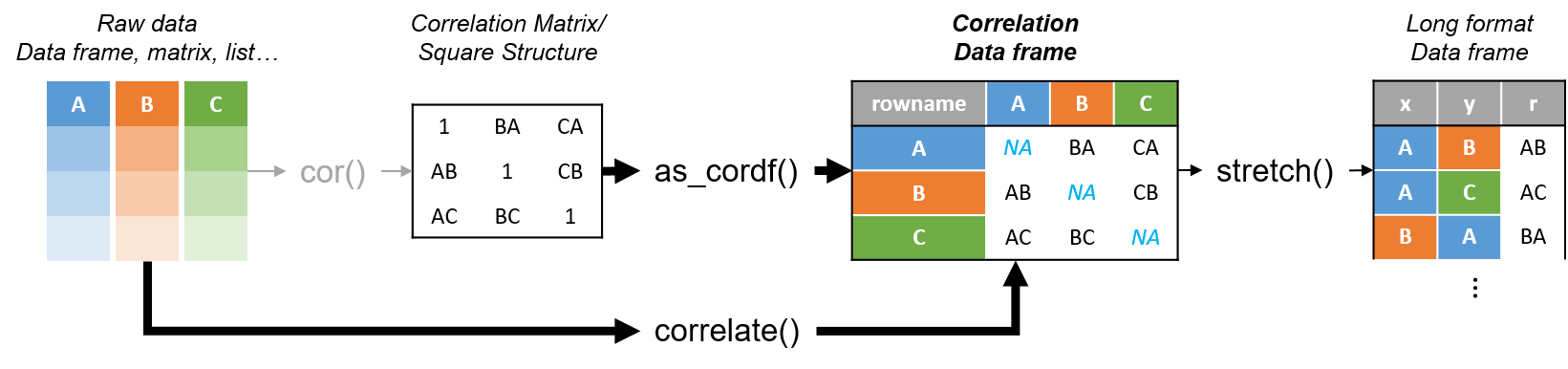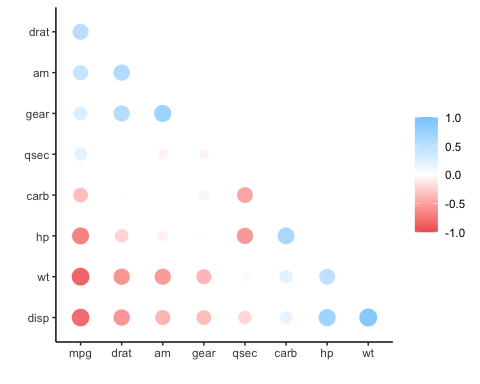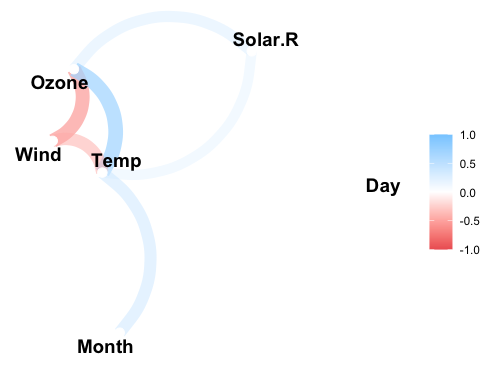corrrcorrr is a package for exploring correlations in R. It focuses on creating and working with data frames of correlations (instead of matrices) that can be easily explored via corrr functions or by leveraging tools like those in the tidyverse. This, along with the primary corrr functions, is represented below:You can install:

• the latest released version from CRAN with
# install.packages("corrr")
• the latest development version from GitHub with
# install.packages("remotes")
# remotes::install_github("tidymodels/corrr")

Using corrr

Using corrr typically starts with correlate(), which acts like the base correlation function cor(). It differs by defaulting to pairwise deletion, and returning a correlation data frame (cor_df) of the following structure:

• A tbl with an additional class, cor_df
• An extra “term” column
• Standardized variances (the matrix diagonal) set to missing values (NA) so they can be ignored.

API

The corrr API is designed with data pipelines in mind (e.g., to use %>% from the magrittr package). After correlate(), the primary corrr functions take a cor_df as their first argument, and return a cor_df or tbl (or output like a plot). These functions serve one of three purposes:

Internal changes (cor_df out):

• shave() the upper or lower triangle (set to NA).
• rearrange() the columns and rows based on correlation strengths.

Reshape structure (tbl or cor_df out):

• focus() on select columns and rows.
• stretch() into a long format.

Output/visualizations (console/plot out):

• fashion() the correlations for pretty printing.
• rplot() the correlations with shapes in place of the values.
• network_plot() the correlations in a network.

Databases and Spark

The correlate() function also works with database tables. The function will automatically push the calculations of the correlations to the database, collect the results in R, and return the cor_df object. This allows for those results integrate with the rest of the corrr API.

Examples

library(MASS)
library(corrr)
set.seed(1)

# Simulate three columns correlating about .7 with each other
mu <- rep(0, 3)
Sigma <- matrix(.7, nrow = 3, ncol = 3) + diag(3)*.3
seven <- mvrnorm(n = 1000, mu = mu, Sigma = Sigma)

# Simulate three columns correlating about .4 with each other
mu <- rep(0, 3)
Sigma <- matrix(.4, nrow = 3, ncol = 3) + diag(3)*.6
four <- mvrnorm(n = 1000, mu = mu, Sigma = Sigma)

# Bind together
d <- cbind(seven, four)
colnames(d) <- paste0("v", 1:ncol(d))

# Insert some missing values
d[sample(1:nrow(d), 100, replace = TRUE), 1] <- NA
d[sample(1:nrow(d), 200, replace = TRUE), 5] <- NA

# Correlate
x <- correlate(d)
class(x)
#>  "cor_df"     "tbl_df"     "tbl"        "data.frame"
x
#> # A tibble: 6 x 7
#>   term        v1      v2      v3      v4       v5      v6
#>   <chr>    <dbl>   <dbl>   <dbl>   <dbl>    <dbl>   <dbl>
#> 1 v1    NA        0.696   0.705   0.0137  0.00906 -0.0467
#> 2 v2     0.696   NA       0.697  -0.0133  0.0221  -0.0338
#> 3 v3     0.705    0.697  NA      -0.0253 -0.0166  -0.0201
#> 4 v4     0.0137  -0.0133 -0.0253 NA       0.452    0.442
#> 5 v5     0.00906  0.0221 -0.0166  0.452  NA        0.425
#> 6 v6    -0.0467  -0.0338 -0.0201  0.442   0.425   NA

NOTE: Previous to corrr 0.4.3, the first column of a cor_df dataframe was named “rowname”. As of corrr 0.4.3, the name of this first column changed to “term”.

As a tbl, we can use functions from data frame packages like dplyr, tidyr, ggplot2:

library(dplyr)

# Filter rows by correlation size
x %>% filter(v1 > .6)
#> # A tibble: 2 x 7
#>   term     v1     v2     v3      v4      v5      v6
#>   <chr> <dbl>  <dbl>  <dbl>   <dbl>   <dbl>   <dbl>
#> 1 v2    0.696 NA      0.697 -0.0133  0.0221 -0.0338
#> 2 v3    0.705  0.697 NA     -0.0253 -0.0166 -0.0201

corrr functions work in pipelines (cor_df in; cor_df or tbl out):

x <- datasets::mtcars %>%
correlate() %>%    # Create correlation data frame (cor_df)
focus(-cyl, -vs, mirror = TRUE) %>%  # Focus on cor_df without 'cyl' and 'vs'
rearrange() %>%  # rearrange by correlations
shave() # Shave off the upper triangle for a clean result
#>
#> Correlation method: 'pearson'
#> Missing treated using: 'pairwise.complete.obs'
#> Registered S3 method overwritten by 'seriation':
#>   method         from
#>   reorder.hclust gclus

fashion(x)
#>   term  mpg drat   am gear qsec carb   hp   wt disp
#> 1  mpg
#> 2 drat  .68
#> 3   am  .60  .71
#> 4 gear  .48  .70  .79
#> 5 qsec  .42  .09 -.23 -.21
#> 6 carb -.55 -.09  .06  .27 -.66
#> 7   hp -.78 -.45 -.24 -.13 -.71  .75
#> 8   wt -.87 -.71 -.69 -.58 -.17  .43  .66
#> 9 disp -.85 -.71 -.59 -.56 -.43  .39  .79  .89
rplot(x)
#> Don't know how to automatically pick scale for object of type noquote. Defaulting to continuous.datasets::airquality %>%
correlate() %>%
network_plot(min_cor = .2)
#>
#> Correlation method: 'pearson'
#> Missing treated using: 'pairwise.complete.obs'